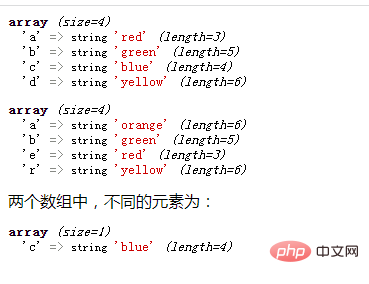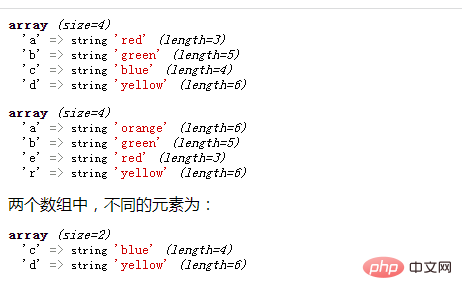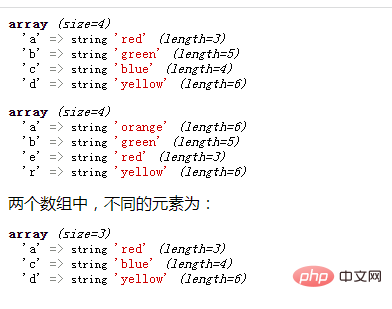# php怎么比较两个数组并返回不同元素php比较两个数组并返回不同元素

• array_diff()函数

• array_diff_key()函数

• array_diff_assoc()函数

1、array_diff()函数--比较数组的键值

array_diff(\$arr1,\$arr2...)函数只比较数组的键值，会返回一个差集数组，差集数组中的元素是存在于被比较的数组\$arr1中，但不存在于其他参数数组\$arr2...中的。

```<?php
\$arr1=array("a"=>"red","b"=>"green","c"=>"blue","d"=>"yellow");
\$arr2=array("a"=>"orange","b"=>"green","e"=>"red","r"=>"yellow");
var_dump(\$arr1);
var_dump(\$arr2);
\$result=array_diff(\$arr1,\$arr2);
echo "两个数组中，不同的元素为：";
var_dump(\$result);
?>```2、array_diff_key()函数--比较数组的键名

array_diff_key(\$arr1,\$arr2...)函数只比较数组的键名，同样返回一个差集数组，差集数组中的元素是存在于被比较的数组\$arr1中，但不存在于其他参数数组\$arr2...中的。

```<?php
\$arr1=array("a"=>"red","b"=>"green","c"=>"blue","d"=>"yellow");
\$arr2=array("a"=>"orange","b"=>"green","e"=>"red","r"=>"yellow");
var_dump(\$arr1);
var_dump(\$arr2);
\$result=array_diff_key(\$arr1,\$arr2);
echo "两个数组中，不同的元素为：";
var_dump(\$result);
?>```3、array_diff_assoc()函数--比较数组的键名和键值

array_diff_assoc(\$arr1,\$arr2...)函数会将数组的键名和键值都进行比较，同样返回一个差集数组，差集元素和array_diff()、array_diff_key()一样会从被比较的数组\$arr1中获取。

```<?php
\$arr1=array("a"=>"red","b"=>"green","c"=>"blue","d"=>"yellow");
\$arr2=array("a"=>"orange","b"=>"green","e"=>"red","r"=>"yellow");
var_dump(\$arr1);
var_dump(\$arr2);
\$result=array_diff_assoc(\$arr1,\$arr2);
echo "两个数组中，不同的元素为：";
var_dump(\$result);
?>```1/1